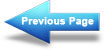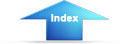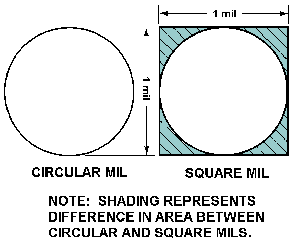Custom SearchElectric CurrentMatter, Energy, and ElectricityConductanceMeasurement of Current

The magnitude of current is measured in AMPERES. A current of one ampere is said to flow when one coulomb of charge passes a point in one second. Remember, one coulomb is equal to the charge of 6.28 x 1018 electrons.

Frequently, the ampere is much too large a unit for measuring current. Therefore, the MILLIAMPERE (mA), one-thousandth of an ampere, or the MICROAMPERE (A), one-millionth of an ampere, is used. The device used to measure current is called an AMMETER and will be discussed in detail in a later module.

Q48.Convert 350 mA to amperes.   Test Yourself

ELECTRICAL RESISTANCE

It is known that the directed movement of electrons constitutes a current flow. It is also known that the electrons do not move freely through a conductor's crystalline structure. Some materials offer little opposition to current flow, while others greatly oppose current flow. This opposition to current flow is known as RESISTANCE (R), and the unit of measure is the OHM. The standard of measure for one ohm is the resistance provided at zero degrees Celsius by a column of mercury having a cross-sectional area of one square millimeter and a length of 106.3 centimeters. A conductor has one ohm of resistance when an applied potential of one volt produces a current of one ampere. The symbol used to represent the ohm is the Greek letter omega (W).

Resistance, although an electrical property, is determined by the physical structure of a material. The resistance of a material is governed by many of the same factors that control current flow. Therefore, in a subsequent discussion, the factors that affect current flow will be used to assist in the explanation of the factors affecting resistance.

Q49.What is the symbol for ohm?   Test Yourself

Factors That Affect Resistance

The magnitude of resistance is determined in part by the "number of free electrons" available within the material. Since a decrease in the number of free electrons will decrease the current flow, it can be said that the opposition to current flow (resistance) is greater in a material with fewer free electrons. Thus, the resistance of a material is determined by the number of free electrons available in a material.

A knowledge of the conditions that limit current flow and, therefore, affect resistance can now be used to consider how the type of material, physical dimensions, and temperature will affect the resistance of a conductor.

TYPE OF MATERIAL. - Depending upon their atomic structure, different materials will have different quantities of free electrons. Therefore, the various conductors used in electrical applications have different values of resistance.

Consider a simple metallic substance. Most metals are crystalline in structure and consist of atoms that are tightly bound in the lattice network. The atoms of such elements are so close together that the electrons in the outer shell of the atom are associated with one atom as much as with its neighbor. (See fig. 1-27 view A). As a result, the force of attachment of an outer electron with an individual atom is practically zero. Depending on the metal, at least one electron, sometimes two, and in a few cases, three electrons per atom exist in this state. In such a case, a relatively small amount of additional electron energy would free the outer electrons from the attraction of the nucleus. At normal room temperature materials of this type have many free electrons and are good conductors. Good conductors will have a low resistance.Figure 1-27. - Atomic spacing in conductors.

If the atoms of a material are farther apart, as illustrated in figure 1-27 view B, the electrons in the outer shells will not be equally attached to several atoms as they orbit the nucleus. They will be attracted by the nucleus of the parent atom only. Therefore, a greater amount of energy is required to free any of these electrons. Materials of this type are poor conductors and therefore have a high resistance.

Silver, gold, and aluminum are good conductors. Therefore, materials composed of their atoms would have a low resistance.

The element copper is the conductor most widely used throughout electrical applications. Silver has a lower resistance than copper but its cost limits usage to circuits where a high conductivity is demanded.

Aluminum, which is considerably lighter than copper, is used as a conductor when weight is a major factor.

Q50.When would silver be used as a conductor in preference to copper?   Test Yourself

EFFECT OF CROSS-SECTIONAL AREA. - Cross-sectional area greatly affects the magnitude of resistance. If the cross-sectional area of a conductor is increased, a greater quantity of electrons are available for movement through the conductor. Therefore, a larger current will flow for a given amount of applied voltage. An increase in current indicates that when the cross-sectional area of a conductor is increased, the resistance must have decreased. If the cross-sectional area of a conductor is decreased, the number of available electrons decreases and, for a given applied voltage, the current through the conductor decreases. A decrease in current flow indicates that when the cross-sectional area of a conductor is decreased, the resistance must have increased. Thus, the RESISTANCE OF A CONDUCTOR IS INVERSELY PROPORTIONAL TO ITS CROSS-SECTIONAL AREA.

The diameter of conductors used in electronics is often only a fraction of an inch, therefore, the diameter is expressed in mils (thousandths of an inch). It is also standard practice to assign the unit circular mil to the cross-sectional area of the conductor. The circular mil is found by squaring the diameter when the diameter is expressed in mils. Thus, if the diameter is 35 mils (0.035 inch), the circular mil area is equal to (35)2 or 1225 circular mils. A comparison between a square mil and a circular mil is illustrated in figure 1-28.Figure 1-28. - Square and circular mils.

EFFECT OF CONDUCTOR LENGTH. - The length of a conductor is also a factor which determines the resistance of a conductor. If the length of a conductor is increased, the amount of energy given up increases. As free electrons move from atom to atom some energy is given off as heat. The longer a conductor is, the more energy is lost to heat. The additional energy loss subtracts from the energy being transferred through the conductor, resulting in a decrease in current flow for a given applied voltage. A decrease in current flow indicates an increase in resistance, since voltage was held constant. Therefore, if the length of a conductor is increased, the resistance increases. THE RESISTANCE OF A CONDUCTOR IS DIRECTLY PROPORTIONAL TO ITS LENGTH.

Q51.Which wire has the least resistance? Wire A-copper, 1000 circular mils, 6 inches long. Wire B-copper, 2000 circular mils, 11 inches long.   Test Yourself

EFFECT OF TEMPERATURE. - Temperature changes affect the resistance of materials in different ways. In some materials an increase in temperature causes an increase in resistance, whereas in others, an increase in temperature causes a decrease in resistance. The amount of change of resistance per unit change in temperature is known as the TEMPERATURE COEFFICIENT. If for an increase in temperature the resistance of a material increases, it is said to have a POSITIVE TEMPERATURE COEFFICIENT. A material whose resistance decreases with an increase in temperature has a NEGATIVE TEMPERATURE COEFFICIENT. Most conductors used in electronic applications have a positive temperature coefficient. However, carbon, a frequently used material, is a substance having a negative temperature coefficient. Several materials, such as the alloys constantan and manganin, are considered to have a ZERO TEMPERATURE COEFFICIENT because their resistance remains relatively constant for changes in temperature.

Q52.Which temperature coefficient indicates a material whose resistance increases as temperature increases?   Test Yourself
Q53.What term describes a material whose resistance remains relatively constant with changes in temperature?   Test YourselfIntegrated Publishing, Inc. - A (SDVOSB) Service Disabled Veteran Owned Small Business